## 回顾分治算法

1）分：取得数组A中的中间数，并将其与n比较
2）治：假设数组为递增数组，若n比中间数小，则在数组左半部分继续递归查找执行“分”步骤
3）组合：由于在数组A中找到n后便直接返回了，因此这一步就无足轻重了

f0=0

f1=1

fi=fi1+fi2(i>1)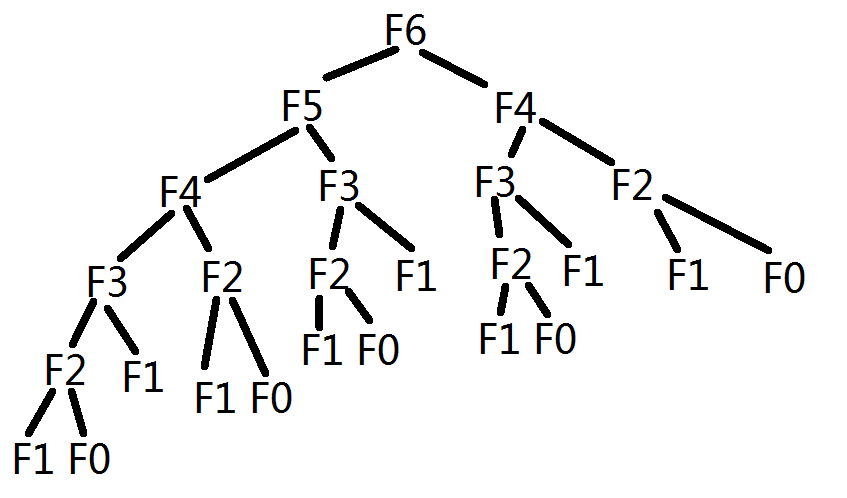Fi=ΦiΦ¯i(5)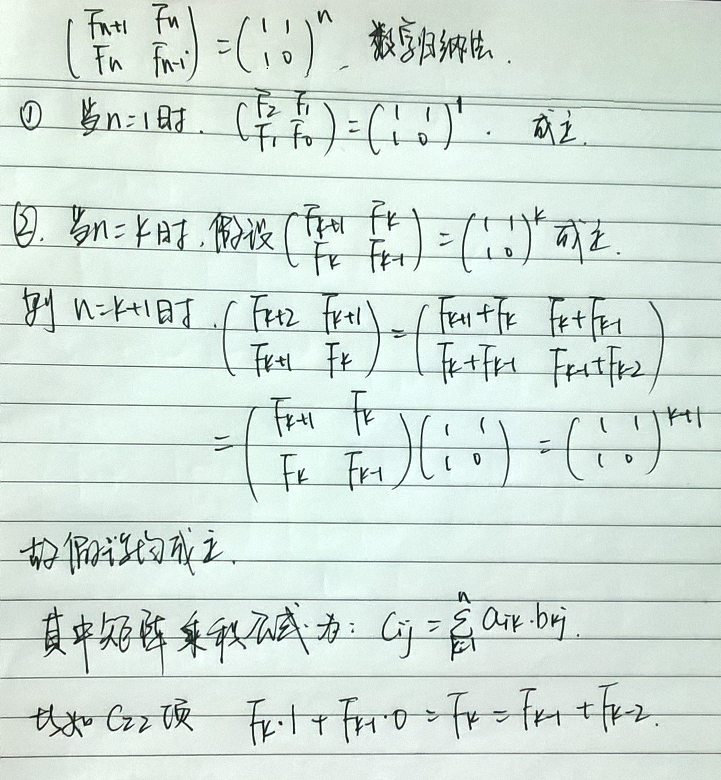## 天 0 1 2 3 4 5 6 7 8 9 10 11 12 13 14 15 16 17 18 股票价格 50 57 65 75 67 60 54 51 48 44 47 43 56 64 71 65 61 73 70 价格波动 0 7 8 18 -8 -7 -6 -3 -3 -4 3 -4 13 10 7 -6 -4 12 -3

A 7 8 18 -8 -7 -6 -3 -3 -4 3 -4 13 10 7 -6 -4 12 -3

## 使用分治思想解决问题

1）完全位于子数组 A[low...mid] 中，因此 lowijmid
2）完全位于子数组 A[mid+1...high] 中，因此 mid<ijhigh
3）跨越中点 mid ，因此 lowimidjhigh

A[low...high] 的一个最大子数组所处的位置必然是这三种情况之一，而且还是这三种情况中所有子数组中和最大者。对于第1种和第2种情况我们可以通过递归来求解最大子数组，对于第三种情况我们可以通过下面伪代码所示来求解。

FIND-MAX-CROSSING-SUBARRAY(A,low,mid,high)
1   left-sum = -10000
2   sum = 0
3   for i = mid downto low
4        sum = sum + A[i]
5        if sum > left-sum
6             left-sum = sum
7             max-left = i
8   right-sum = -10000
9   sum = 0
10  for j = mid + 1 to high
11       sum = sum + A[i]
12       if sum > right-sum
13            right-sum = sum
14            max-right = j
15   return (max-left, max-right, left-sum + right-sum)

#include <iostream>
#include <cstdio>

using namespace std;

int const n=18;
int A[n]={7,8,18,-8,-7,-6,-3,-3,-4,3,-4,13,10,7,-6,-4,12,-3};
int B;
int low,high,mid;
int max_left,max_right;
int sum;

void find_max_crossing_subarray(int A[],int low,int mid,int high);

int main()
{
find_max_crossing_subarray(A,0,7,15);
for(int i=0;i<3;i++)
{
printf("%d ",B[i]);
}
return 0;
}

void find_max_crossing_subarray(int A[],int low,int mid,int high)
{
int left_sum=-10000;
sum=0;
for(int i=mid;i>=low;i--)
{
sum=sum+A[i];
if(sum>left_sum)
{
left_sum=sum;
max_left=i;
}
}
int right_sum=-10000;
sum=0;
for(int j=mid+1;j<=high;j++)
{
sum=sum+A[j];
if(sum>right_sum)
{
right_sum=sum;
max_right=j;
}
}
B=max_left;
B=max_right;
B=left_sum+right_sum;
}


(midlow+1)+(highmid)=highlow+1=n

FIND-MAXIMUM-SUBARRAY(A,low,high)
1   if high==low
2        return (low,high,A[low])
4        (left-low,left-high,left-sum)=FIND-MAXIMUM-SUBARRAY(A,low,mid)
5        (right-low,right-high,right-sum)=FIND-MAXIMUM-SUBARRAY(A,mid+1,high)
6        (cross-low,cross-high,cross-sum)=FIND-MAX-CROSSING-SUBARRAY(A,low,mid,high)
7        if left-sum>=right-sum and left-sum>=cross-sum)
return (left-low,left-high,left-sum)
8        else if(right-sum>=left-sum and right-sum>=cross-sum)
return (right-low,right-high,right-sum)
9        else return (cross-low,cross-high,cross-sum)

## 分析分治算法和渐近记号中的省略问题

T(1)=Θ(1)

T(n)=Θ(1)+2T(n/2)+Θ(n)+Θ(1)=2T(n/2)+Θ(n)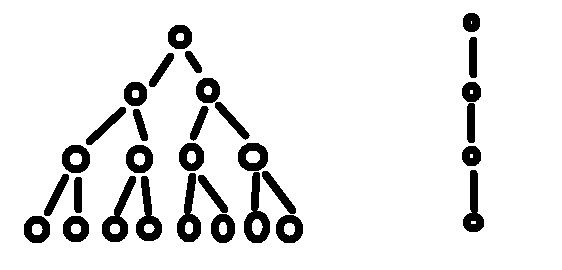## 借助递归树求解递归式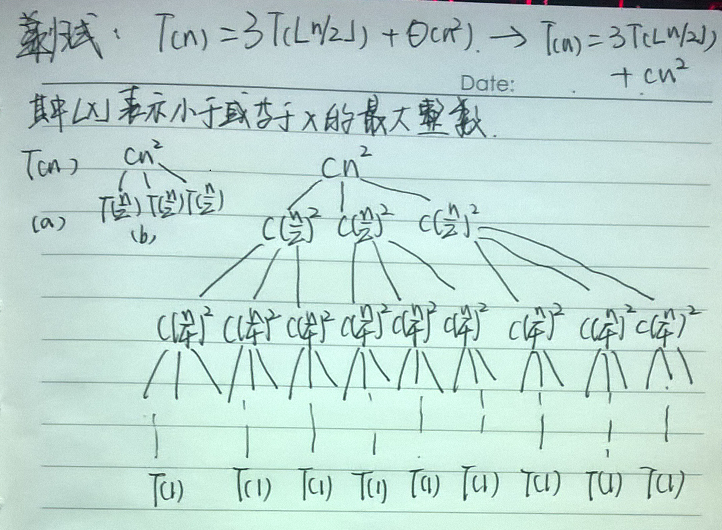1）我们要关注的更应该是解的上界，因为我们知道舍入对求解递归式没有影响，因此可以将 Θ(n2) 写成 cn2 ，且为该递归式创建了如下递归树。

2）我们还将 n 假定为2的幂，这样所有子问题的规模均为正数。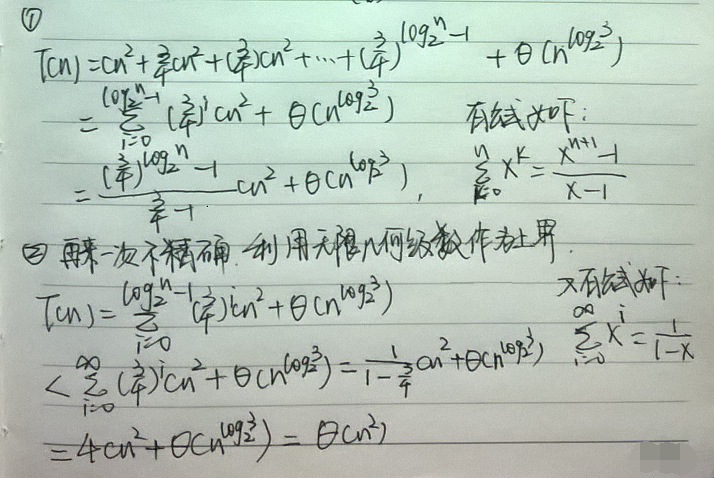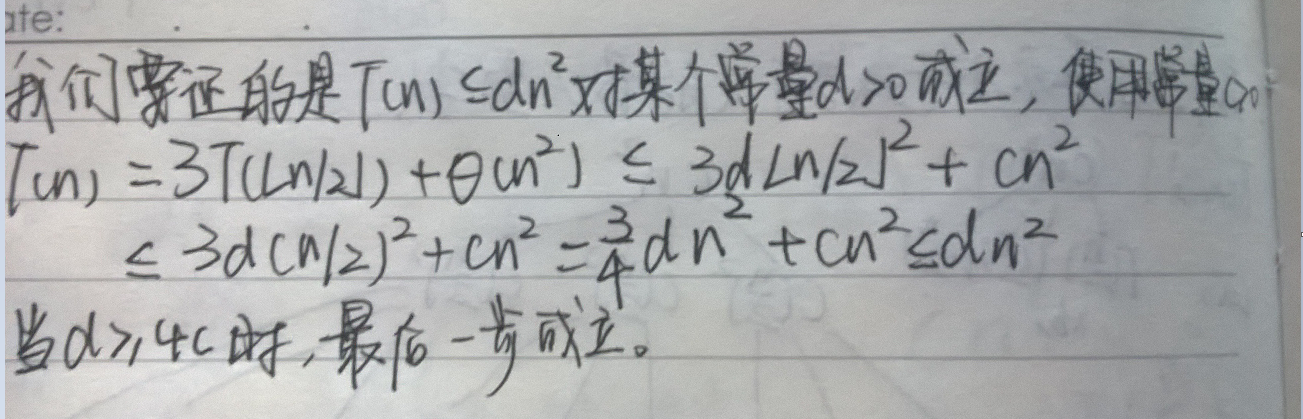http://blog.csdn.net/nomasp### 评论(0)

PoolMan
2019/08/18
0
0

2019/09/19
0
0
「leetcode」买卖股票的最佳时机I、II、III、IV、含手续费、含冷冻期

2019/09/28
0
0

Clever Liu
2016/03/27
0
0
LeetCode与剑指Offer接替思路及代码总结

309.Best Time to Buy and Sell Stock with Cooldown 题目： 给出n天股票的价格，求买卖的最大利润。 规则： 可以买卖多次。但买入新股票前必须卖出旧股票 如果在第i天卖出股票，那第i+1天不...

2019/04/27
0
0

00-Java 面试准备

54
0
OSChina 周二乱弹 —— 大王（@罗马的王）颜值制霸Osc社区

Osc乱弹歌单（2020）请戳（这里） 【今日歌曲】 @巴拉迪维 ：Lunik的单曲《Seeing You Soar》 I hope you’re smiling，When seeing me soar. #今日歌曲推荐# 《Seeing You Soar》- Lunik 手...

83
0
wordcount代码

1.写出map类 public class WCMapper extends Mapper<LongWritable,Text,Text,LongWritable>{ @Override protected void map(LongWritable key,Text value,Context context)throws IOExcepti......

59
0

Chunk-Oriented Processing不是处理 step 的唯一方法。 考虑下面的一个场景，如果你仅仅需要调用一个存储过程，你可以在 ItemReader 中实现这个调用，然后在存储过程完成调用后返回 null。这...

honeymoose

67
0
Linux日志分析

1. Linux日志文件的类型 2. 系统服务日志 2.1 syslogd的简介 2.2 syslogd的配置和使用 2.3 日志的安全性设置 2.4 远程日志记录服务 3. 日志的轮替 3.1 logrotate简介 3.2 logrotate的配置 3....

JiaMing

67
0